# multcompare

Multiple comparison test

## Syntax

``c = multcompare(stats)``
``c = multcompare(stats,Name,Value)``
``````[c,m] = multcompare(___)``````
``````[c,m,h] = multcompare(___)``````
``````[c,m,h,gnames] = multcompare(___)``````

## Description

example

````c = multcompare(stats)` returns a matrix `c` of the pairwise comparison results from a multiple comparison test using the information contained in the `stats` structure. `multcompare` also displays an interactive graph of the estimates and comparison intervals. Each group mean is represented by a symbol, and the interval is represented by a line extending out from the symbol. Two group means are significantly different if their intervals are disjoint; they are not significantly different if their intervals overlap. If you use your mouse to select any group, then the graph will highlight all other groups that are significantly different, if any.```

example

````c = multcompare(stats,Name,Value)` specifies options using one or more name-value arguments. For example, you can specify the confidence interval, or the type of critical value to use in the multiple comparison test.```
``````[c,m] = multcompare(___)``` also returns a matrix, `m`, which contains estimated values of the means (or whatever statistics are being compared) for each group and the corresponding standard errors. You can use any of the previous syntaxes.```
``````[c,m,h] = multcompare(___)``` also returns a handle, `h`, to the comparison graph.```

example

``````[c,m,h,gnames] = multcompare(___)``` also returns a cell array, `gnames`, which contains the names of the groups.```

## Examples

collapse all

Load the `carsmall` data set.

`load carsmall`

The data contains miles per gallon (`MPG`) measurements for different makes and models of cars, grouped by the country of origin (`Origin`) and other vehicle characteristics.

Perform a one-way analysis of variance (ANOVA) to see if the MPG values of the cars are different depending on the country of origin.

`[p,t,stats] = anova1(MPG,Origin);`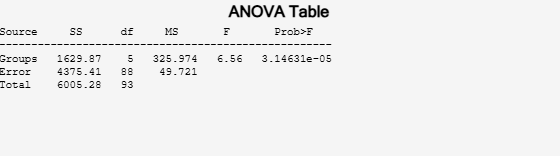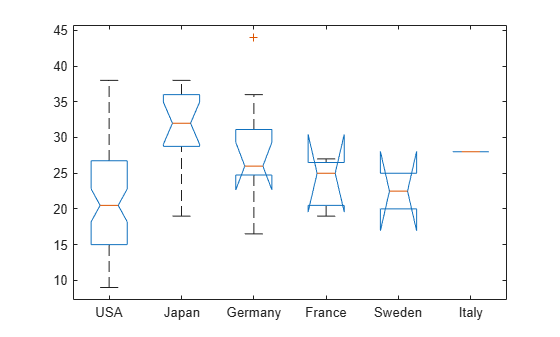The small p-value (value in the column `Prob>F`) indicates that group mean differences are significant. However, the ANOVA results do not indicate which groups have different means. You can perform pairwise comparisons using a multiple comparison test to identify the groups that have significantly different means.

Perform a multiple comparison test of the group means.

`[c,m,h,gnames] = multcompare(stats);`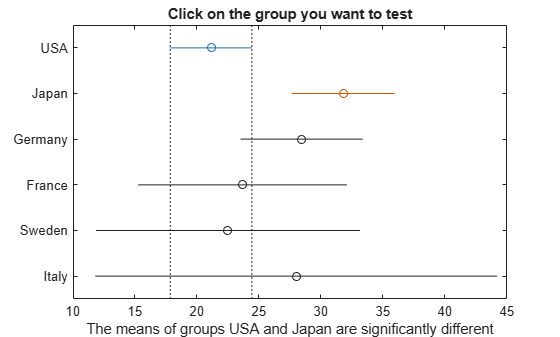`multcompare` displays the estimates with comparison intervals around them. You can click the graph of any country to compare its mean to those of other countries.

Display the mean estimates, standard errors, and corresponding group names in a table.

```tbl = array2table(m,"RowNames",gnames, ... "VariableNames",["Mean","Standard Error"])```
```tbl=6×2 table Mean Standard Error ______ ______________ USA 21.133 0.88141 Japan 31.8 1.8206 Germany 28.444 2.3504 France 23.667 4.0711 Sweden 22.5 4.986 Italy 28 7.0513 ```

Perform a multiple comparison test against a control group using Dunnett's test, and compare the results to the pairwise comparison results from Tukey’s honestly significant difference procedure.

Load the `carsmall` data set.

`load carsmall`

The data contains miles per gallon (`MPG`) measurements for different makes and models of cars, grouped by the country of origin (`Origin`) and other vehicle characteristics.

Perform a one-way ANOVA to compare the mileage of the cars across the groups defined by their countries of origin.

`[~,~,stats] = anova1(MPG,Origin,"off");`

Display the names of the groups.

`stats.gnames`
```ans = 6x1 cell {'USA' } {'Japan' } {'Germany'} {'France' } {'Sweden' } {'Italy' } ```

According to the multiple comparison results for all distinct pairs of groups in the Multiple Comparisons of Group Means example, USA and Japan have significantly different means. The example uses the default test, Tukey’s honestly significant difference procedure.

Compare the group means against a control group by using Dunnett's test.

Specify CriticalValueType as "`dunnett"` to perform Dunnett's test. `multcompare` selects the first group (USA) as the control group by default. You can select a different control group by using the ControlGroup name-value argument.

`[results,~,~,gnames] = multcompare(stats,"CriticalValueType","dunnett");`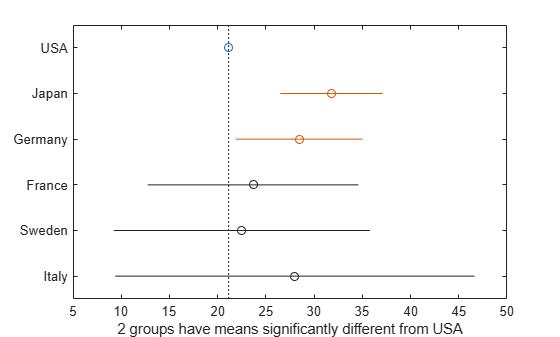In the figure, the blue circle indicates the mean of the control group. The red circles and bars represent the means and confidence intervals for the groups with significantly different means from the mean of the control group. Note that the red bars do not cross the dotted vertical line representing the mean of the control group. Groups that do not have significantly different means appear in grey.

Dunnett's test identifies that two groups, Japan and Germany, have means that are significantly different from the mean of the USA (control group). Note that the default procedure (Tukey’s honestly significant difference procedure) did not identify Germany in the Multiple Comparisons of Group Means example. The difference in the results is related to the different levels of conservativeness in the two comparison tests. Dunnett's test is less conservative than the default procedure because the test considers only the comparisons against a control group. The default procedure performs pairwise comparisons for all distinct pairs of groups.

Display the multiple comparison results and the corresponding group names in a table.

```tbl = array2table(results,"VariableNames", ... ["Group","Control Group","Lower Limit","Difference","Upper Limit","P-value"]); tbl.("Group") = gnames(tbl.("Group")); tbl.("Control Group") = gnames(tbl.("Control Group"))```
```tbl=5×6 table Group Control Group Lower Limit Difference Upper Limit P-value ___________ _____________ ___________ __________ ___________ _________ {'Japan' } {'USA'} 5.3649 10.667 15.969 4.727e-06 {'Germany'} {'USA'} 0.73151 7.3116 13.892 0.022346 {'France' } {'USA'} -8.3848 2.5339 13.453 0.97912 {'Sweden' } {'USA'} -11.905 1.3672 14.64 0.99953 {'Italy' } {'USA'} -11.76 6.8672 25.495 0.86579 ```

```load popcorn popcorn```
```popcorn = 6×3 5.5000 4.5000 3.5000 5.5000 4.5000 4.0000 6.0000 4.0000 3.0000 6.5000 5.0000 4.0000 7.0000 5.5000 5.0000 7.0000 5.0000 4.5000 ```

The data is from a study of popcorn brands and popper types (Hogg 1987). The columns of the matrix `popcorn` are brands (Gourmet, National, and Generic). The rows are popper types oil and air. The first three rows correspond to the oil popper, and the last three rows correspond to the air popper. In the study, researchers popped a batch of each brand three times with each popper. The values are the yield in cups of popped popcorn.

Perform a two-way ANOVA. Also compute the statistics that you need to perform a multiple comparison test on the main effects.

`[~,~,stats] = anova2(popcorn,3,"off")`
```stats = struct with fields: source: 'anova2' sigmasq: 0.1389 colmeans: [6.2500 4.7500 4] coln: 6 rowmeans: [4.5000 5.5000] rown: 9 inter: 1 pval: 0.7462 df: 12 ```

The `stats` structure includes

• The mean squared error (`sigmasq`)

• The estimates of the mean yield for each popcorn brand (`colmeans`)

• The number of observations for each popcorn brand (`coln`)

• The estimate of the mean yield for each popper type (`rowmeans`)

• The number of observations for each popper type (`rown`)

• The number of interactions (`inter`)

• The p-value that shows the significance level of the interaction term (`pval`)

• The error degrees of freedom (`df`).

Perform a multiple comparison test to see if the popcorn yield differs between pairs of popcorn brands (columns).

`c1 = multcompare(stats);`
```Note: Your model includes an interaction term. A test of main effects can be difficult to interpret when the model includes interactions. ```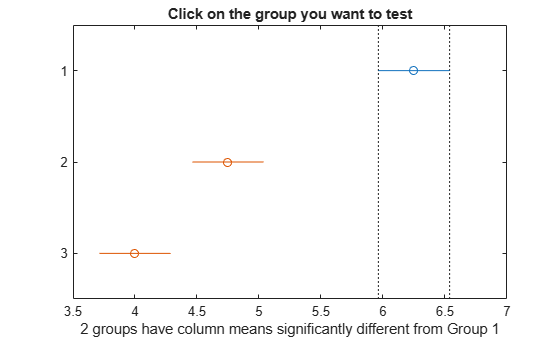The figure shows the multiple comparisons of the means. By default, the group 1 mean is highlighted and the comparison interval is in blue. Because the comparison intervals for the other two groups do not intersect with the intervals for the group 1 mean, they are highlighted in red. This lack of intersection indicates that both means are different than group 1 mean. Select other group means to confirm that all group means are significantly different from each other.

Display the multiple comparison results in a table.

```tbl1 = array2table(c1,"VariableNames", ... ["Group A","Group B","Lower Limit","A-B","Upper Limit","P-value"])```
```tbl1=3×6 table Group A Group B Lower Limit A-B Upper Limit P-value _______ _______ ___________ ____ ___________ __________ 1 2 0.92597 1.5 2.074 4.1188e-05 1 3 1.676 2.25 2.824 6.1588e-07 2 3 0.17597 0.75 1.324 0.011591 ```

The first two columns of `c1` show the groups that are compared. The fourth column shows the difference between the estimated group means. The third and fifth columns show the lower and upper limits for 95% confidence intervals for the true mean difference. The sixth column contains the p-value for a hypothesis test that the corresponding mean difference is equal to zero. All p-values are very small, which indicates that the popcorn yield differs across all three brands.

Perform a multiple comparison test to see the popcorn yield differs between the two popper types (rows).

`c2 = multcompare(stats,"Estimate","row");`
```Note: Your model includes an interaction term. A test of main effects can be difficult to interpret when the model includes interactions. ```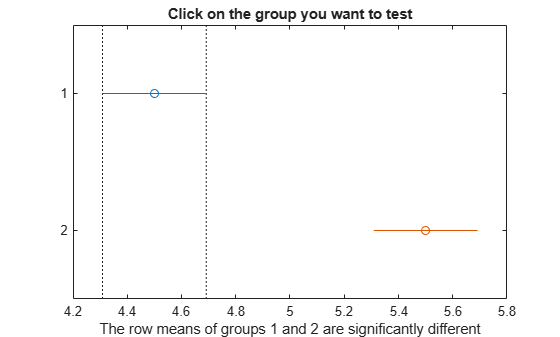```tbl2 = array2table(c2,"VariableNames", ... ["Group A","Group B","Lower Limit","A-B","Upper Limit","P-value"])```
```tbl2=1×6 table Group A Group B Lower Limit A-B Upper Limit P-value _______ _______ ___________ ___ ___________ __________ 1 2 -1.3828 -1 -0.61722 0.00010037 ```

The small p-value indicates that the popcorn yield differs between the two popper types (air and oil). The figure shows the same results. The disjoint comparison intervals indicate that the group means are significantly different from each other.

```y = [52.7 57.5 45.9 44.5 53.0 57.0 45.9 44.0]'; g1 = [1 2 1 2 1 2 1 2]; g2 = ["hi" "hi" "lo" "lo" "hi" "hi" "lo" "lo"]; g3 = ["may" "may" "may" "may" "june" "june" "june" "june"];```

`y` is the response vector and `g1`, `g2`, and `g3` are the grouping variables (factors). Each factor has two levels, and every observation in `y` is identified by a combination of factor levels. For example, observation `y(1)` is associated with level 1 of factor `g1`, level `hi` of factor `g2`, and level `may` of factor `g3`. Similarly, observation `y(6)` is associated with level 2 of factor `g1`, level `hi` of factor `g2`, and level `june` of factor `g3`.

Test if the response is the same for all factor levels. Also compute the statistics required for multiple comparison tests.

```[~,~,stats] = anovan(y,{g1 g2 g3},"Model","interaction", ... "Varnames",["g1","g2","g3"]);```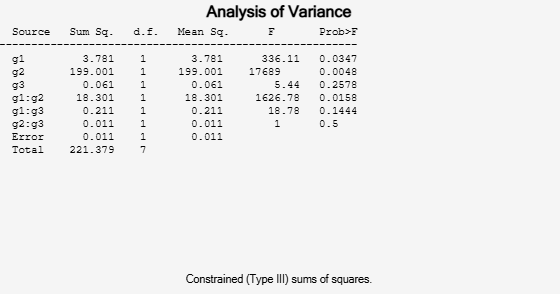The p-value of 0.2578 indicates that the mean responses for levels `may` and `june` of factor `g3` are not significantly different. The p-value of 0.0347 indicates that the mean responses for levels `1` and `2` of factor `g1` are significantly different. Similarly, the p-value of 0.0048 indicates that the mean responses for levels `hi` and `lo` of factor `g2` are significantly different.

Perform a multiple comparison test to find out which groups of factors `g1` and `g2` are significantly different.

`[results,~,~,gnames] = multcompare(stats,"Dimension",[1 2]);`You can test the other groups by clicking on the corresponding comparison interval for the group. The bar you click on turns to blue. The bars for the groups that are significantly different are red. The bars for the groups that are not significantly different are gray. For example, if you click on the comparison interval for the combination of level `1` of `g1` and level `lo` of `g2`, the comparison interval for the combination of level `2` of `g1` and level `lo` of `g2` overlaps, and is therefore gray. Conversely, the other comparison intervals are red, indicating significant difference.

Display the multiple comparison results and the corresponding group names in a table.

```tbl = array2table(results,"VariableNames", ... ["Group A","Group B","Lower Limit","A-B","Upper Limit","P-value"]); tbl.("Group A")=gnames(tbl.("Group A")); tbl.("Group B")=gnames(tbl.("Group B"))```
```tbl=6×6 table Group A Group B Lower Limit A-B Upper Limit P-value ______________ ______________ ___________ _____ ___________ _________ {'g1=1,g2=hi'} {'g1=2,g2=hi'} -6.8604 -4.4 -1.9396 0.027249 {'g1=1,g2=hi'} {'g1=1,g2=lo'} 4.4896 6.95 9.4104 0.016983 {'g1=1,g2=hi'} {'g1=2,g2=lo'} 6.1396 8.6 11.06 0.013586 {'g1=2,g2=hi'} {'g1=1,g2=lo'} 8.8896 11.35 13.81 0.010114 {'g1=2,g2=hi'} {'g1=2,g2=lo'} 10.54 13 15.46 0.0087375 {'g1=1,g2=lo'} {'g1=2,g2=lo'} -0.8104 1.65 4.1104 0.07375 ```

The `multcompare` function compares the combinations of groups (levels) of the two grouping variables, `g1` and `g2`. For example, the first row of the matrix shows that the combination of level `1` of `g1` and level `hi` of `g2` has the same mean response values as the combination of level `2` of `g1` and level `hi` of `g2`. The p-value corresponding to this test is `0.0272`, which indicates that the mean responses are significantly different. You can also see this result in the figure. The blue bar shows the comparison interval for the mean response for the combination of level `1` of `g1` and level `hi` of `g2`. The red bars are the comparison intervals for the mean response for other group combinations. None of the red bars overlap with the blue bar, which means the mean response for the combination of level `1` of `g1` and level `hi` of `g2` is significantly different from the mean response for other group combinations.

## Input Arguments

collapse all

Test data, specified as a structure. You can create a structure using one of the following functions:

`multcompare` does not support multiple comparisons using `anovan` output for a model that includes random or nested effects. The calculations for a random effects model produce a warning that all effects are treated as fixed. Nested models are not accepted.

Data Types: `struct`

### Name-Value Arguments

Specify optional pairs of arguments as `Name1=Value1,...,NameN=ValueN`, where `Name` is the argument name and `Value` is the corresponding value. Name-value arguments must appear after other arguments, but the order of the pairs does not matter.

Example: `Alpha=0.01,CriticalValueType="bonferroni",Display="off"` computes the Bonferroni critical values, conducts the hypothesis tests at the 1% significance level, and omits the interactive display.

Before R2021a, use commas to separate each name and value, and enclose `Name` in quotes.

Example: `"Alpha",0.01,"CriticalValueType","bonferroni","Display","off"`

Significance level of the multiple comparison test, specified as a scalar value in the range (0,1). The value specified for `Alpha` determines the 100 × (1 – α) confidence levels of the intervals returned in the matrix `c` and in the figure.

Example: `"Alpha",0.01`

Data Types: `single` | `double`

Flag to compute a critical value for Dunnett's test using an approximate method, specified as logical `1` (`true`) or `0` (`false`).

The `multcompare` function finds a critical value for Dunnett's test by integrating the multivariate t distribution. The computation can be slow for multiway (n-way) ANOVA if n is large. To speed up the computation, you can use an approximate method () by specifying `Approximate` as `true`. The approximate method involves randomness. If you want to reproduce the results, set the random seed by using the `rng` function before calling `multcompare`.

The default value is `true` if the source of `stats` is `anovan`. Otherwise, the default value is `false`.

This argument is valid only when `CriticalValueType` is `"dunnett"`.

Example: `"Approximate",true`

Data Types: `logical`

Index of the control group for Dunnett's test, specified as a positive integer value.

Specify one of the groups compared by the `multcompare` function as the control group. Assume that you specify `ControlGroup` as `idx`. This table shows the control group value, which depends on the source of `stats`.

Source of `stats`Control Group
`anova1`

`multcompare` uses `stats.gnames(idx)`, the group corresponding to `stats.means(idx)`, as the control group.

`anova2`

`multcompare` uses the group corresponding to `stats.colmeans(idx)` (if `Estimate` is `"column"` (default)) or `stats.rowmeans(idx)` (if `Estimate` is `"row"`) as the control group.

`anovan`

If you specify `Dimension` as `d`, then `multcompare` uses `stats.grpnames{d}(idx)` as the control group.

`aoctool`

`multcompare` uses `stats.gnames(idx)` as the control group.

`friedman`

`multcompare` uses the group corresponding to `stats.meanranks(idx)` as the control group.

`kruskalwallis`

`multcompare` uses `stats.gnames(idx)`, the group corresponding to `stats.meanranks(idx)`, as the control group.

This argument is valid only when `CriticalValueType` is `"dunnett"`.

Example: `"ControlGroup",3`

Data Types: `single` | `double`

Type of the critical value to use for the multiple comparison test, specified as one of the following.

ValueDescription
`"lsd"`Fisher's least significant difference procedure
`"dunnett"`Dunnett's test
`"tukey-kramer"` or `"hsd"` (default)Tukey’s honestly significant difference procedure
`"dunn-sidak"`Dunn & Sidák’s approach
`"bonferroni"`Bonferroni method
`"scheffe"`Scheffe’s procedure

The table lists the critical value types in order of conservativeness, from least to most conservative. Each test provides a different level of protection against the multiple comparison problem.

• `"lsd"` does not provide any protection.

• `"dunnett"` provides protection for comparisons against a control group.

• `"tukey-kramer"`, `"dunn-sidak"`, and `"bonferroni"` provide protection for pairwise comparisons.

• `"scheffe"` provides protection for pairwise comparisons and comparisons of all linear combinations of the estimates.

Example: `"CriticalValueType","bonferroni"`

Data Types: `string` | `char`

Display toggle, specified as either `"on"` or `"off"`. If you specify `"on"`, then `multcompare` displays a graph of the estimates and their comparison intervals. If you specify `"off"`, then `multcompare` omits the graph.

Example: `"Display","off"`

Data Types: `string` | `char`

Dimension or dimensions over which to calculate the population marginal means, specified as a positive integer value, or a vector of such values. If you specify `CriticalValueType` as `"dunnett"`, then you can specify only one dimension.

This argument is valid only when you create the input structure `stats` using the function `anovan`.

For example, if you specify `Dimension` as `1`, then `multcompare` compares the means for each value of the first grouping variable, adjusted by removing effects of the other grouping variables as if the design were balanced. If you specify `Dimension` as `[1,3]`, then `multcompare` computes the population marginal means for each combination of the first and third grouping variables, removing effects of the second grouping variable. If you fit a singular model, some cell means may not be estimable and any population marginal means that depend on those cell means will have the value `NaN`.

Population marginal means are described by Milliken and Johnson (1992) and by Searle, Speed, and Milliken (1980). The idea behind population marginal means is to remove any effect of an unbalanced design by fixing the values of the factors specified by `Dimension`, and averaging out the effects of other factors as if each factor combination occurred the same number of times. The definition of population marginal means does not depend on the number of observations at each factor combination. For designed experiments where the number of observations at each factor combination has no meaning, population marginal means can be easier to interpret than simple means ignoring other factors. For surveys and other studies where the number of observations at each combination does have meaning, population marginal means may be harder to interpret.

Example: `"Dimension",[1,3]`

Data Types: `single` | `double`

Estimates to be compared, specified as an allowable value. The allowable values for `Estimate` depend on the function used to generate the input structure `stats`, according to the following table.

FunctionValues
`anova1`

None. `multcompare` ignores this argument and always compares the group means.

`anova2`

Either `"column"` to compare column means or `"row"` to compare row means.

`anovan`

None. `multcompare` ignores this argument and always compares the population marginal means as specified by the `Dimension` name-value argument.

`aoctool`

`"slope"`, `"intercept"`, or `"pmm"` to compare slopes, intercepts, or population marginal means, respectively. If the analysis of covariance model does not include separate slopes, then `"slope"` is not allowed. If the model does not include separate intercepts, then no comparisons are possible.

`friedman`

None. `multcompare` ignores this argument and always compares the average column ranks.

`kruskalwallis`

None. `multcompare` ignores this argument and always compares the average group ranks.

Example: `"Estimate","row"`

Data Types: `string` | `char`

## Output Arguments

collapse all

Matrix of multiple comparison results, returned as an p-by-6 matrix of scalar values, where p is the number of pairs of groups. Each row of the matrix contains the result of one paired comparison test. Columns 1 and 2 contain the indices of the two samples being compared. Column 3 contains the lower confidence interval, column 4 contains the estimate, and column 5 contains the upper confidence interval. Column 6 contains the p-value for the hypothesis test that the corresponding mean difference is not equal to 0.

For example, suppose one row contains the following entries.

`2.0000 5.0000 1.9442 8.2206 14.4971 0.0432`

These numbers indicate that the mean of group 2 minus the mean of group 5 is estimated to be 8.2206, and a 95% confidence interval for the true difference of the means is [1.9442, 14.4971]. The p-value for the corresponding hypothesis test that the difference of the means of groups 2 and 5 is significantly different from zero is 0.0432.

In this example the confidence interval does not contain 0, so the difference is significant at the 5% significance level. If the confidence interval did contain 0, the difference would not be significant. The p-value of 0.0432 also indicates that the difference of the means of groups 2 and 5 is significantly different from 0.

Matrix of the estimates, returned as a matrix of scalar values. The first column of `m` contains the estimated values of the means (or whatever statistics are being compared) for each group, and the second column contains their standard errors.

Handle to the figure containing the interactive graph, returned as a handle. The title of this graph contains instructions for interacting with the graph, and the x-axis label contains information about which means are significantly different from the selected mean. If you plan to use this graph for presentation, you may want to omit the title and the x-axis label. You can remove them using interactive features of the graph window, or you can use the following commands.

```title("") xlabel("")```

Group names, returned as a cell array of character vectors. Each row of `gnames` contains the name of a group.

collapse all

### Multiple Comparison Tests

Analysis of variance compares the means of several groups to test the hypothesis that they are all equal, against the general alternative that they are not all equal. Sometimes this alternative may be too general. You may need information about which pairs of means are significantly different, and which are not. A multiple comparison test can provide this information.

When you perform a simple t-test of one group mean against another, you specify a significance level that determines the cutoff value of the t-statistic. For example, you can specify the value `alpha` = `0.05` to ensure that when there is no real difference, you will incorrectly find a significant difference no more than 5% of the time. When there are many group means, there are also many pairs to compare. If you applied an ordinary t-test in this situation, the `alpha` value would apply to each comparison, so the chance of incorrectly finding a significant difference would increase with the number of comparisons. Multiple comparison procedures are designed to provide an upper bound on the probability that any comparison will be incorrectly found significant.

### Null and Alternative Hypotheses

The `multcompare` function examines different sets of null hypotheses (H0) and alternative hypotheses (H1) depending on the type of critical value specified by the `CriticalValueType` name-value argument.

• Dunnett's test (`CriticalValueType` is `"dunnett"`) performs multiple comparisons against a control group. Therefore, the null and alternative hypotheses for a comparison against the control group are

where mi and m0 are estimates for group i and the control group, respectively. The function examines H0 and H1 multiple times for all noncontrol groups.

• For the other tests, `multcompare` performs multiple pairwise comparisons for all distinct pairs of groups. The null and alternative hypotheses of a pairwise comparison between group i and j are

 Hochberg, Y., and A. C. Tamhane. Multiple Comparison Procedures. Hoboken, NJ: John Wiley & Sons, 1987.

 Milliken, G. A., and D. E. Johnson. Analysis of Messy Data, Volume I: Designed Experiments. Boca Raton, FL: Chapman & Hall/CRC Press, 1992.

 Searle, S. R., F. M. Speed, and G. A. Milliken. “Population marginal means in the linear model: an alternative to least-squares means.” American Statistician. 1980, pp. 216–221.

 Dunnett, Charles W. “A Multiple Comparison Procedure for Comparing Several Treatments with a Control.” Journal of the American Statistical Association, vol. 50, no. 272, Dec. 1955, pp. 1096–121.

 Krishnaiah, Paruchuri R., and J. V. Armitage. "Tables for multivariate t distribution." Sankhyā: The Indian Journal of Statistics, Series B (1966): 31-56.